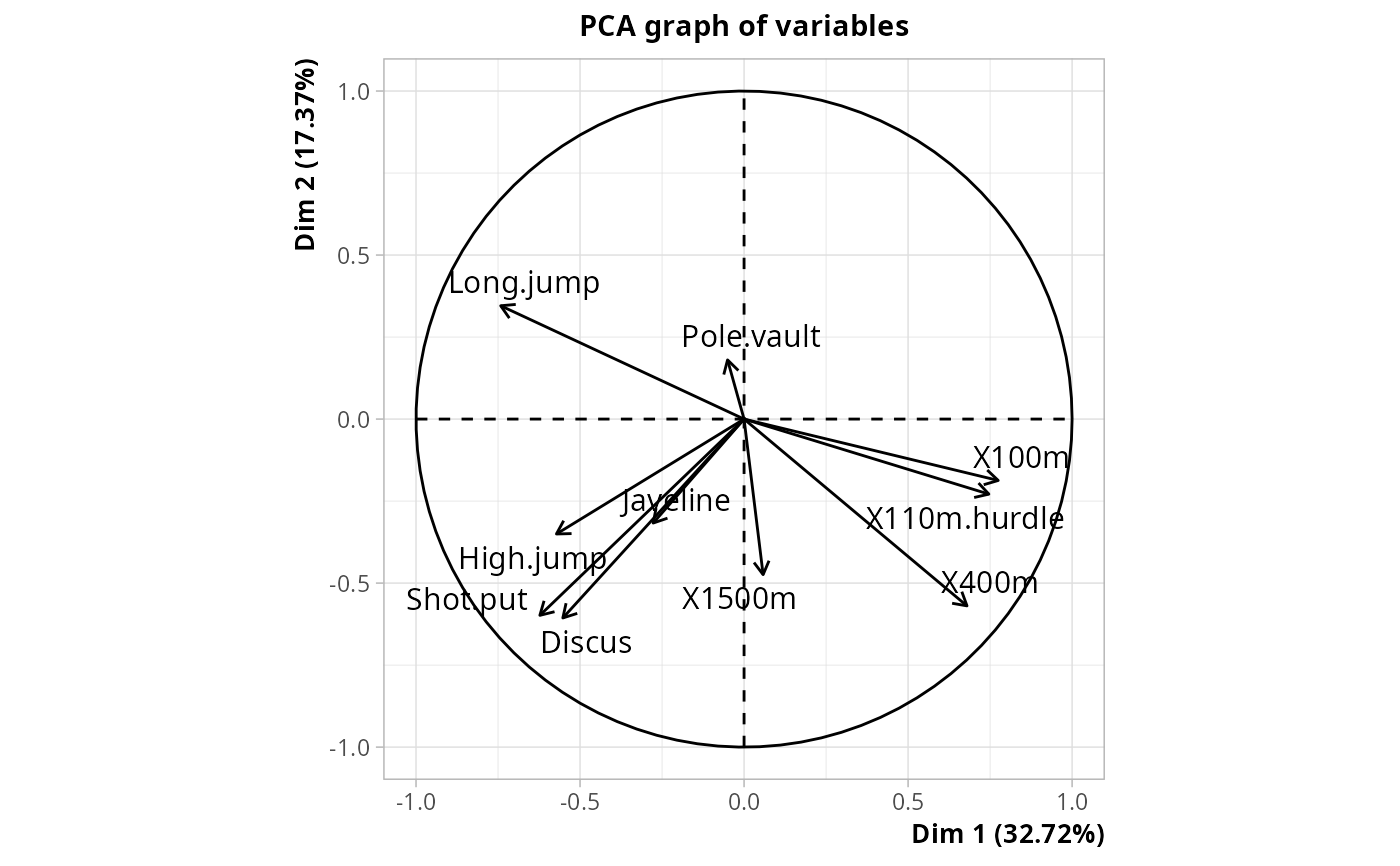Generalized Principal Component Analysis

gPCA(X, row.w = NULL, col.w = NULL, center = FALSE, scale = FALSE, tol = 1e-07)

## Arguments

X

data frame of active variables

row.w

numeric vector of row weights. If NULL (default), a vector of 1 for uniform row weights is used.

col.w

numeric vector of column weights. If NULL (default), a vector of 1 for uniform column weights is used.

center

logical. If TRUE, variables are centered (default is FALSE).

scale

logical. If TRUE, variables are scaled to unit variance (default is FALSE).

tol

a tolerance threshold for null eigenvalues (a value less than tol times the first one is considered as null)

## Details

Generalized PCA is basically a PCA with the possibility to specify row weights (i.e. "masses") and variable weights (i.e. the "metric"), and to choose whether to center and scale the variables. This flexibility makes it the building block of many variants of PCA, such as Correspondence Analysis and Multiple Correspondence Analysis.

Generalized PCA is also known as "biweighted PCA", "duality diagram" or "generalized singular value decomposition".

## Value

An object of class PCA from FactoMineR package

Nicolas Robette

## Examples

library(FactoMineR)
data(decathlon)
res <- gPCA(decathlon[,1:10], center = TRUE, scale = TRUE)
plot(res, choix = "var")